# Find Matrice Determinant help

#### Description

The determinant command in the matrices section of QuickMath allows you to find the determinant of any square matrix (a matrix which has the same number of rows and columns).

The determinant of a square matrix A can be calculated in terms of its elements ai,j and the determinants of the smaller matrices Ai,j.

For example, if A is a 3 x 3 matrix, then its determinant can be found as follows :

det(A) = a1,1 |A1,1| - a1,2 |A1,2| + a1,3 |A1,3|

where ai,j is the element of A at row i, column j and Ai,j is the matrix constructed from A by removing row i and column j.

The determinant of a 2 x 2 matrix

a b
c d

can be easily memorized, rather than calculated from scratch. It is

a d - b c.

To use the determinant command, simply go to the determinant page, type in your matrix and hit the "Determinant " button. Your question will be automatically answered by computer and the reply will be shown in your browser within a few seconds. The answer will be given in two forms : a 'natural' form, which mimics the row and column layout normally used for matrices, and an 'input' form, which is the form required for the entry of matrices into QuickMath. The input form of a solution can be copied and pasted into a matrix input field, so you can use the result from one calculation in another calculation without having to re-type it.

#### Examples

Here are some examples illustrating the types of matrices you can use the determinant command on and the results which QuickMath will return.

 Matrix Result a, b c, d -(b c) + a d 1, 2 3, 4 -2 5, 7, 3, 4 8, 9, 2, 3 1, 5, 3, 8 3, 6, 9, 0 -36

# PROPERTIES OF DETERMINANTS

Row transformations can be used to show that for the linear system

ax+ by= m

ex+ dy = n,

the matrix of coefficients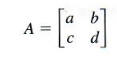has inverse matrix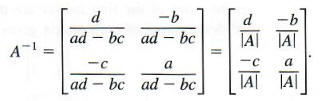Thus, if |A| = 0, A-1 does not exist. It can be shown that this idea extends to n x n matrices in general. This provides a way to determine whether a system of equations has a single solution without actually solving the system. The properties of determinants given in this section make it easier to decide quickly if a determinant is zero or to evaluate a nonzero determinant more quickly.

The value of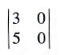is 3*0 - 5*0 = 0. Also, expanded about the second row,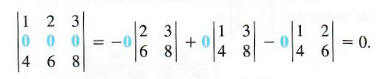The fact that the matrix for each of these determinants has a row or column of zeros leads to a determinant of 0. These examples suggest the following result.

DETERMINANT PROPERTY 1: If every element in a row or column of a matrix is 0, then the determinant equals 0.

To prove Property I, expand the determinant about the 0 row or column. Each term of this expansion has a 0 factor, so each term is 0. For example,Example 1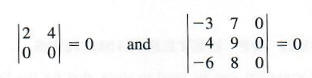Suppose the matrixis rewritten as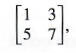so the rows of the first matrix are the columns of the second matrix. Evaluating the determinant of each matrix gives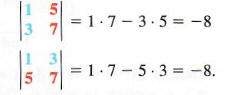The two determinants are the same, suggesting the next property of determinants.

DETERMINANT PROPERTY 2: If corresponding rows and columns of a matrix are interchanged, the determinant is not changed.

Example 2

Given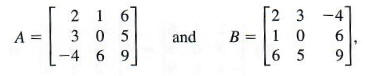by Property 2, since the rows of the first matrix are the columns of the second,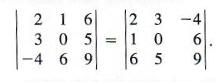Exchanging the rows of the matrix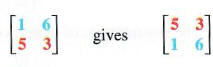While the first determinant isthe second determinant is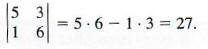The second determinant is the negative of the first. This suggests the third property of determinants.

DETERMINANT PROPERTY 3: Interchanging two rows (or columns) of a matrix reverses the sign of the determinant.

Example 3

(a) Interchange the two columns of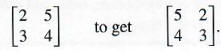By Property 3, since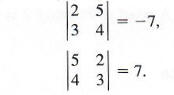thenMultiplying each element of the second row of the matrixby -5 gives the new matrix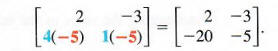The determinants of these two matrices are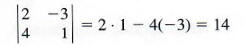andSince -70 = -5(14), the new determinant is -5 times the original determinant. The next property generalizes this idea.

DETERMINANT PROPERTY 4: If every element of a row (or column) of a matrix is multiplied by the real number k, then the determinant of the new matrix is k times the determinant of the original matrix.

Example 4

By Property 4, ifthen |A| = -2*|B|, since the first column of matrix A is -2 times the first column of matrix B.

The matrix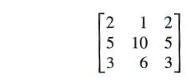has first and third columns that are identical. Expanding the determinant about the second column givesThis result suggests the next property.

DETERMINANT PROPERTY 5:  The determinant of a matrix with two identical rows (or columns) equals 0.

Example 5
Since two rows are identical, the determinant of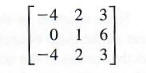equals 0

If we multiply each element of the second row of the matrixby 3 and add the result to the first row, we get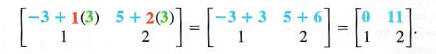Verify that the determinant of the new matrix is the same as the determinant of the original matrix, -11. This idea, which is generalized below, is perhaps the most useful property of determinants presented in this section.

DETERMINANT PROPERTY 6: The determinant of a matrix is unchanged if a multiple of a row (or column) of the matrix is added to the corresponding elements of another row (or column).

Example 6

Multiply each element of the first column of the matrix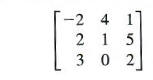by 3, and add the results to the third column to get the new matrix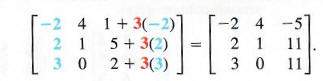By Property 6, the determinants of these two matrices are equal. Verify that each determinant equals 37

The following examples show how the properties of determinants can be used to simplify the calculation of determinants,

Example 7

Without expanding, show that the determinant of the following matrix is zero.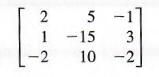Each element in the second column equals -5 times the corresponding element in the third column. Multiply the elements of the third column by 5 and then add the results to the corresponding elements in the second column to get a matrix with an equivalent determinant,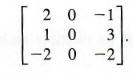By Property 1, the determinant of this matrix is zero.

Example 8

EvaluateUse Property 6 to change the first row (any row or column could be used) of the matrix to a row in which every element but one is zero. Begin by multiplying the elements of the second column by -2 and adding the results to the first column, replacing the first column with this new column. The result is a 0 for the first element in the first row.